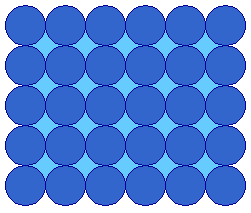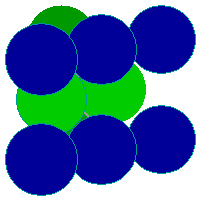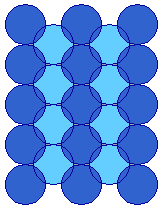# 1.2: Surface Structures: fcc Metals

•• Contributed by Roger Nix
• Senior Lecturer (Physical Chemistry, School of Biological & Chemical Sciences) at Queen Mary, University of London

Many of the technologically most important metals possess the fcc structure, e.g., the catalytically important precious metals (Pt, Rh, Pd) all exhibit an fcc structure. The low index faces of this system are the most commonly studied of surfaces: as we shall see they exhibit a range of

• Surface symmetry
• Surface atom coordination
• Surface reactivity

## I. The fcc (100) Surface

The (100) surface is that obtained by cutting the fcc metal parallel to the front surface of the fcc cubic unit cell - this exposes a surface (the atoms in blue) with an atomic arrangement of 4-fold symmetryfcc unit cell (100) face

The diagram below shows the conventional birds-eye view of the (100) surface - this is obtained by rotating the preceding diagram through 45° to give a view which emphasises the 4-fold (rotational) symmetry of the surface layer atoms.The tops of the second layer atoms are just visible through the holes in the first layer, but would not be accessible to molecules arriving from the gas phase.

Exercise $$\PageIndex{1}$$

What is the coordination number of the surface layer atoms on the fcc(100) surface ?

The coordination number of the surface layer atoms is 8

Rationale: Each surface atom has four nearest neighbors in the 1st layer, and another four in the layer immediately below ; a total of 8. This contrasts with the CN of metal atoms in the bulk of the solid which is 12 for a fcc metal.

There are several other points worthy of note :

1. All the surface atoms are equivalent
2. The surface is relatively smooth at the atomic scale
3. The surface offers various adsorption sites for molecules which have different local symmetries and lead to different coordination geometries - specifically there are:
• On-top sites (above a single metal atom)
• Bridging sites, between two atoms
• Hollow sites, between four atoms

Depending upon the site occupied, an adsorbate species (with a single point of attachments to the surface) is therefore likely to be bonded to either one, two or four metal atoms.

## II. The fcc(110) surface

The (110) surface is obtained by cutting the fcc unit cell in a manner that intersects the x and y axes but not the z-axis - this exposes a surface with an atomic arrangement of 2-fold symmetry.fcc unit cell (110) face

The diagram below shows the conventional birds-eye view of the (110) surface - emphasizing the rectangular symmetry of the surface layer atoms. The diagram has been rotated such that the rows of atoms in the first atomic layer now run vertically, rather than horizontally as in the previous diagram.It is clear from this view that the atoms of the topmost layer are much less closely packed than on the (100) surface - in one direction (along the rows) the atoms are in contact i.e. the distance between atoms is equal to twice the metallic(atomic) radius, but in the orthogonal direction there is a substantial gap between the rows.

This means that the atoms in the underlying second layer are also, to some extent, exposed at the surface(110) surface plane, e.g. Cu(110)

The preceding diagram illustrates some of those second layer atoms, exposed at the bottom of the troughs.

In this case, the determination of atomic coordination numbers requires a little more careful thought : one way to double-check your answer is to remember that the CN of atoms in the bulk of the fcc structure is 12, and then to subtract those which have been removed from above in forming the surface plane.

Exercise $$\PageIndex{2}$$

What is the coordination number of the topmost layer atoms on the fcc(110) surface?

The coordination number of the topmost layer atoms is 7

Rationale: Each surface atom has two nearest neighbours in the 1st layer, and another four in the layer immediately below, and one directly below it in the third layer ; this gives a total of 7.

To confirm this consider those that have been removed from the layers above - clearly there would have been 4 nearest neighbours in the layer immediately above the surface layer ( equivalent to the four in the layer immediately below ). In addition, there would have been one nearest neighbour directly above each surface atom ( equivalent to the one directly below in the third layer ). Hence, 7 (present) + 5 (removed) = 12 - which is correct !

If we compare this coordination number with that obtained for the (100) surface, it is worth noting that the surface atoms on a more open ("rougher") surface have a lower CN; this has important implications when it comes to the chemical reactivity of surfaces.

Do the atoms in the second layer have the bulk coordination ?

No - the fact that they are clearly exposed (visible) at the surface implies that they have a lower CN than they would in the bulk.

Exercise $$\PageIndex{3}$$

What is the coordination number of these second layer atoms on the fcc(110) surface ?

The coordination number of the second layer atoms is 11

Rationale: The atoms in the second layer are only missing one atom from their complete coordination shell ( the atom that would have been directly above them ) i.e. they have CN = (12-1) = 11

In summary, we can note that

1. All first layer surface atoms are equivalent, but second layer atoms are also exposed
2. The surface is atomically rough, and highly anisotropic
3. The surface offers a wide variety of possible adsorption sites, including :
• On-top sites
• Short bridging sites between two atoms in a single row
• Long bridging sites between two atoms in adjacent rows
• Higher coordination sites ( in the troughs )

## III. The fcc (111) surface

The (111) surface is obtained by cutting the fcc metal in such a way that the surface plane intersects the x-, y- and z- axes at the same value - this exposes a surface with an atomic arrangement of 3-fold ( apparently 6-fold, hexagonal ) symmetry. This layer of surface atoms actually corresponds to one of the close-packed layers on which the fcc structure is based.fcc unit cell (111) face

The diagram below shows the conventional birds-eye view of the (111) surface - emphasizing the hexagonal packing of the surface layer atoms. Since this is the most efficient way of packing atoms within a single layer, they are said to be "close-packed".(111) surface plane, e.g. Pt(111)

Exercise $$\PageIndex{4}$$

What is the coordination number of the surface layer atoms on the fcc(111) surface?

The coordination number of the surface layer atoms is 9

Rationale: Each surface atom has six nearest neighbours in the 1st layer, and another three in the layer immediately below ; a total of 9.

The following features are worth noting ;

1. All surface atoms are equivalent and have a relatively high CN
2. The surface is almost smooth at the atomic scale
3. The surface offers the following adsorption sites :
• On-top sites
• Bridging sites, between two atoms
• Hollow sites, between three atoms

## IV. How do these surfaces intersect in irregular-shaped samples

Flat surfaces of single crystal samples correspond to a single Miller Index plane and, as we have seen, each individual surface has a well-defined atomic structure. It is these flat surfaces that are used in most surface science investigations, but it is worth a brief aside to consider what type of surfaces exist for an irregular shaped sample (but one that is still based on a single crystal). Such samples can exhibit facets corresponding to a range of different Miller Index planes. This is best illustrated by looking at the diagrams below.Figure: (left) an angled corner. (right) a spherical tip. From the BALSAC picture gallery of Prof. K. Hermann, Fritz-Haber-Institut, Berlin

## Summary

Depending upon how an fcc single crystal is cleaved or cut, flat surfaces of macroscopic dimensions which exhibit a wide range of structural characteristics may be produced. The single crystal surfaces discussed here ( (100), (110) & (111) ) represent only the most frequently studied surface planes of the fcc system - however, they are also the most commonly occurring surfaces on such metals and the knowledge gained from studies on this limited selection of surfaces goes a long way in propagating the development of our understanding of the surface chemistry of these metals. For further information on other fcc metal surfaces you should take a look at Section 1.8 which includes a brief description of high index fcc surfaces with illustrative examples.

## Contributors

Roger Nix (Queen Mary, University of London)